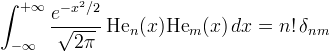﻿OrthogonalPolynomials.HermiteHe MethodComputes the value of a (statisticians') Hermite polynomial.

Namespace:  Meta.Numerics.Functions
Assembly:  Meta.Numerics (in Meta.Numerics.dll) Version: 4.1.4Syntax
```public static double HermiteHe(
int n,
double x
)```

#### Parameters

n
Type: SystemInt32
The order, which must be non-negative.
x
Type: SystemDouble
The argument.

#### Return Value

Type: Double
The value Hen(x).Exceptions
ExceptionCondition
ArgumentOutOfRangeExceptionn is negative.Remarks

Hermite polynomials are orthogonal on the interval (-∞,+∞) with a weight function equal to the standard normal probability distribution.Their ortho-normality relation makes them a useful basis for expressing perturbations around a normal distribution.

Physicists' Hermite polynomials (see HermiteH(Int32, Double)) are related to statisticians' Hermite polynomials via Hn(x) = 2nHn(x √2).See Also